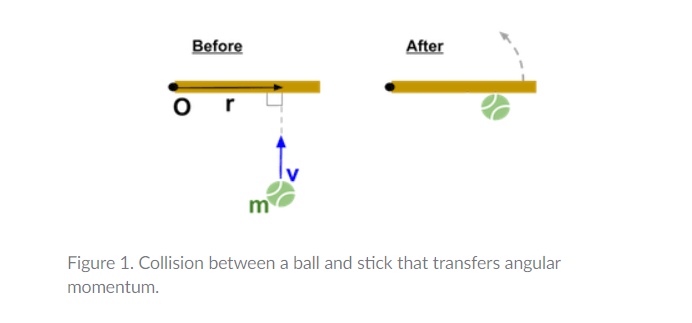# Can an object moving in a straight line, have angular momentum?

An object moving in a straight line (having linear momentum) can have angular momentum.

For example, let’s say we throw a ball at one end of a stick (see Figure 1). The stick can pivot around point O. When the ball hits the stick, the stick rotates.

If the system of the ball and stick has no net external torque, the only way the stick could get angular momentum is from the ball during the collision. Thus, the ball must initially have some angular momentum.

Hence, in this case, we can say that an object moving in a straight line (having linear momentum) can have angular momentum.

## How to find the angular momentum of an object moving in a straight line?we throw a ball at one end of a stick. The stick can pivot around point O. When the ball hits the stick, the stick rotates.

In figure 1, we can see that a ball of mass m is moving in a straight line with linear velocity v. It hits a stick and the stick pivots around point O at the other end of it and rotates. r is the shortest distance between this point O and the point where the ball hits the stick.

The ball’s angular momentum about point O before the collision is calculated using the formula: Lball​=mvr

Hence for this kind of case, we can say that the angular momentum of an object moving in a straight line can be calculated using the formula L=mvr